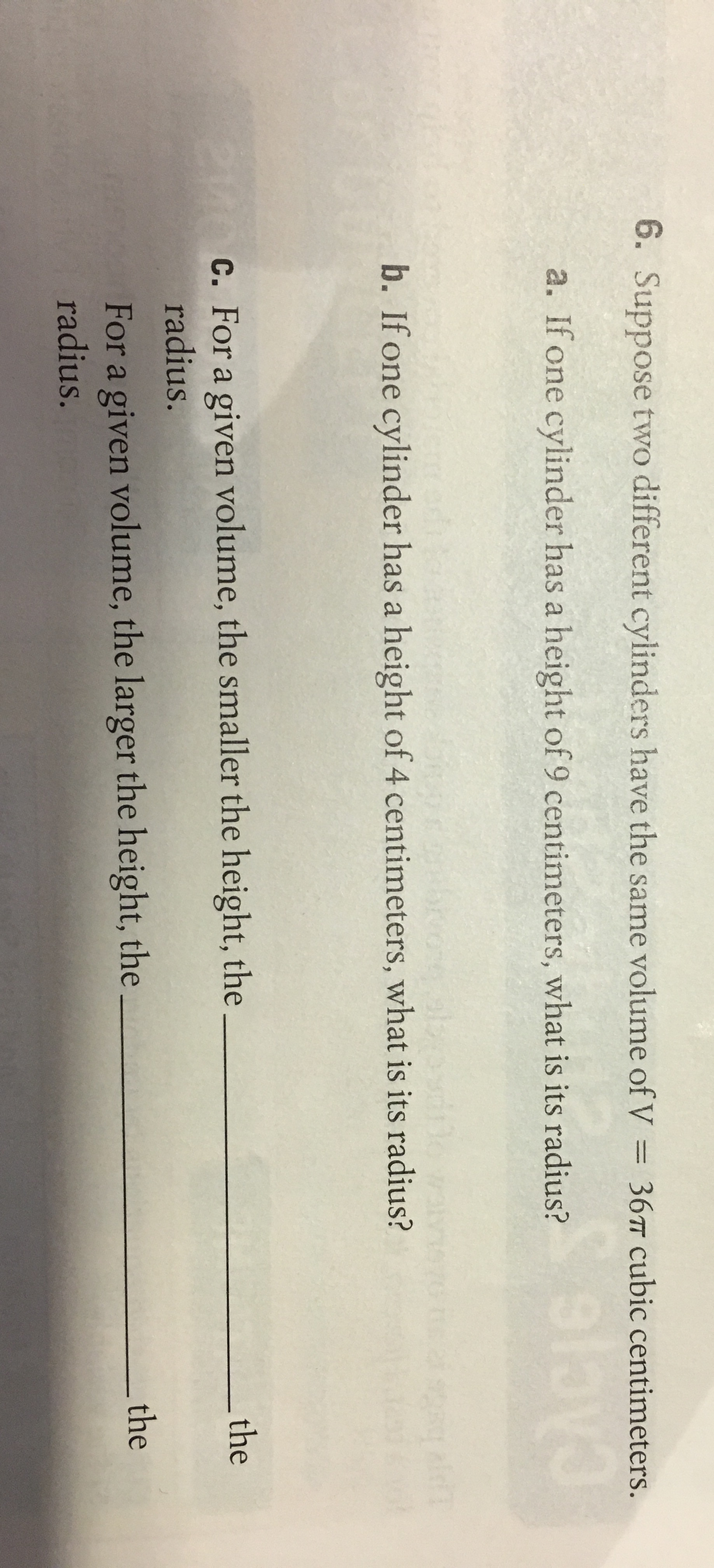6. Suppose two different cylinders have the same volume of V 36 cubic centimetersa. If one cylinder has a height of 9 centimeters, what is its radius?b. If one cylinder has a height of 4 centimeters, what is its radius?c. For a given volume, the smaller the height, thetheradius.For a given volume, the larger the height, theradius.the

Questionhelp_outlineImage Transcriptionclose6. Suppose two different cylinders have the same volume of V 36 cubic centimeters a. If one cylinder has a height of 9 centimeters, what is its radius? b. If one cylinder has a height of 4 centimeters, what is its radius? c. For a given volume, the smaller the height, the the radius. For a given volume, the larger the height, the radius. the fullscreen
Step 1

Application of volume formula for a vylinder.

Step 2

(a) Substituting the given values of V and height h, we obtain r to be 2 cm.

Step 3

(b) Substituting the given values of V an...

Want to see the full answer?

See Solution

Want to see this answer and more?

Our solutions are written by experts, many with advanced degrees, and available 24/7

See Solution
Tagged in

Applications of Mathematics